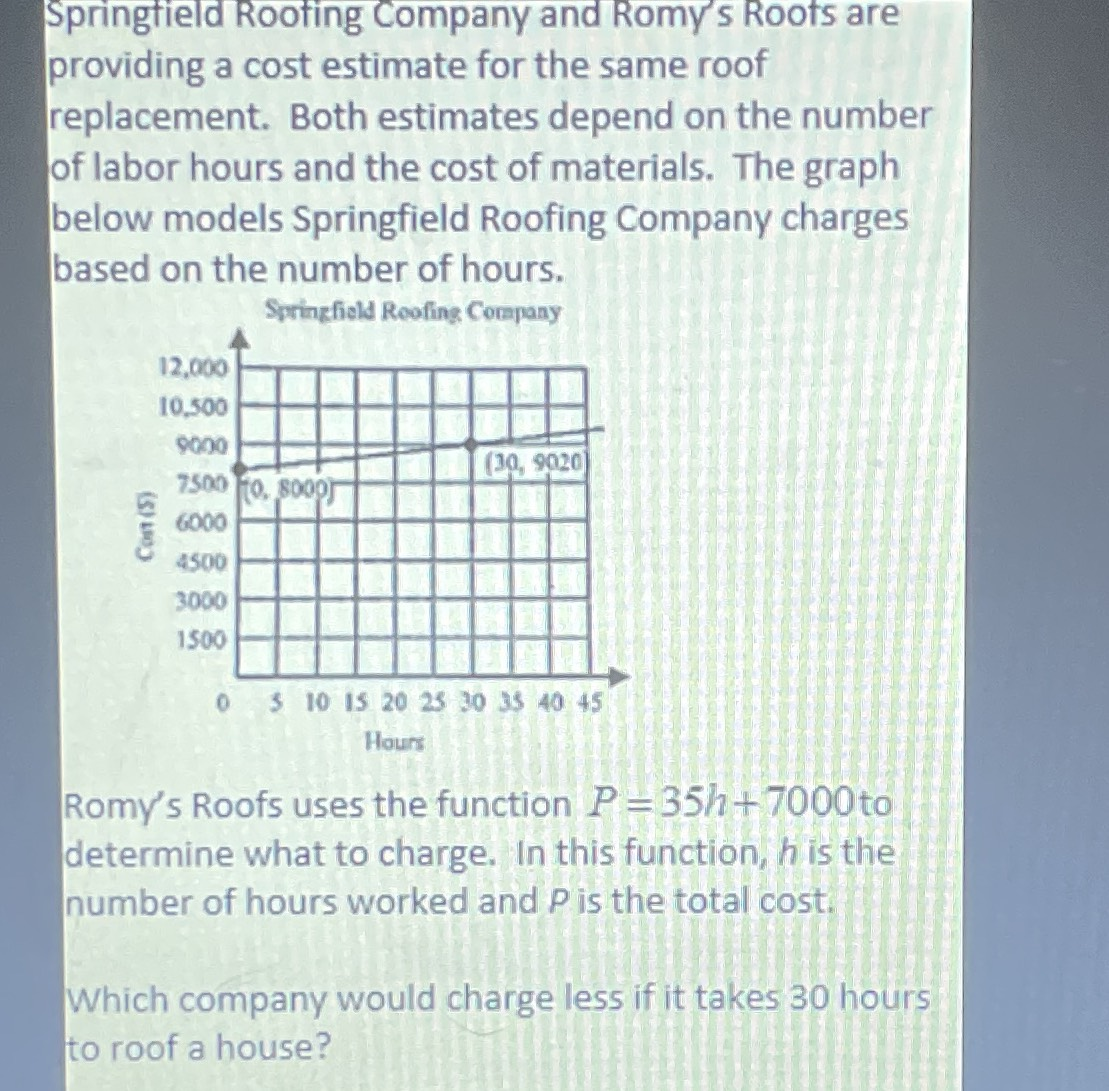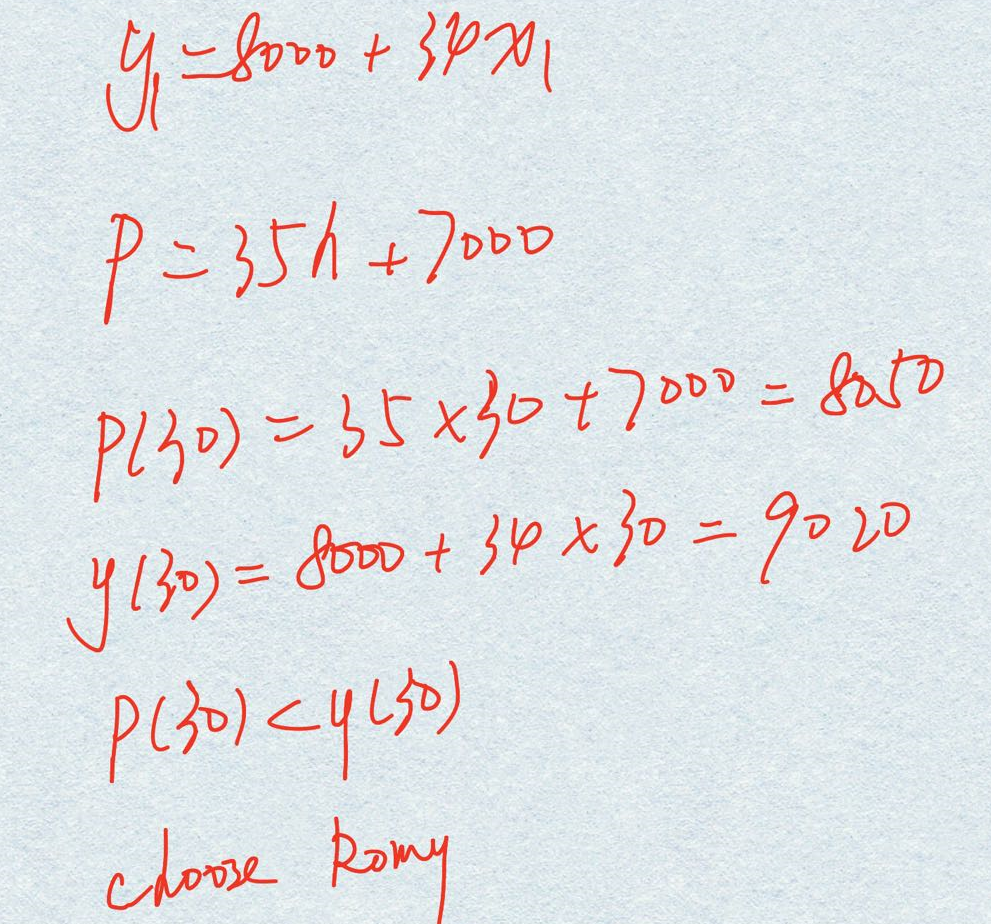### Still have math questions?

Algebra
QuestionSpringfield Rooting Company and Romy's Roots are providing a cost estimate for the same roof replacement. Both estimates depend on the number of labor hours and the cost of materials. The graph below models Springfield Roofing Company charges based on the number of hours.

Romy's Roofs uses the function $$P = 35 h + 7000$$ to determine what to charge. In this function, $$h$$ is the number of hours worked and $$P$$ is the total cost. Which company would charge less if it takes $$30$$ hours to roof a house?